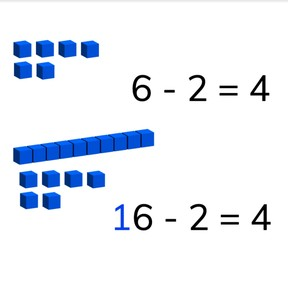Subtraction to 20 using simplification

# Subtraction to 20 using simplification8,000 schools use Gynzy92,000 teachers use Gynzy1,600,000 students use Gynzy

## General

Students learn that they can split a subtraction problem into tens and ones and use the subtraction problem with ones as a first step to subtract with tens.

1.OA.C

## Relevance

It is important to learn this to help make subtraction easier and faster to do.

## Introduction

Start with a few counting exercises counting up to 20. Ask students to count quantities and to explain how they counted. They can skip count in 2s, 3s, and 5s. Then they are asked to decompose numbers to 20 to 10 and the second number. An example is to decompose 13 into 10 and 3.

## Development

Show students a set of subtraction problems that use simplification. Ask students if they notice anything about the subtraction problems. They should notice that the difference between the two problems is 10. Namely that 10 is added to the first number (minuend) and to the difference. These subtraction problems are related. Tell students that you can sometimes solve a subtraction problem with tens faster if you first solve the subtraction problem without the tens. You can use that difference as a first step. If you know 6 - 2 = 4, then you can quickly determine that 16 - 2 = 14. Practice this with MAB blocks on the interactive whiteboard. Drag the blocks that you take away to the trash can, so you can solve the subtraction problem. Ask students how many blocks are left. Emphasize that they don't need to recalculate the subtraction problem, because they can use the first step they already solved. 10 is added to the subtraction problem, so 10 is also added to the difference. Check if students are able to solve a subtraction problem without blocks on the interactive whiteboard as well by using the example of chewing gum. First with 6 - 1 and then with 16 - 1. Do a few more subtraction problems with the students, but without visual support. They can imagine the MAB blocks in their head to help imagine the ten that is added to the second step of the subtraction problem.

Check that students understand subtraction to 20 using simplification by asking the following questions:
- 7 - 2 = 5, what is 17 - 2?
- 14 - 3 = 11. 14 - 13 = 11. How do you know this?
- 9 - 7 = 2, which subtraction problem can you also easily solve now?

## Guided practice

Students start with a subtraction problem in which they get visual support for their simplification. They are then asked to solve the first step as well as the final subtraction problem. Finally they are asked to do so with no visual support.

## Closing

Repeat why it is useful to learn how to subtract using simplification. It helps to make subtraction easier and faster. If you know the subtraction problem without tens, you also know the difference with tens. Check that students understand by doing two subtraction problems as a class, one with visual support and one without visual support.

## Teaching tips

Students who have difficulty can be supported by the use of a rekenrek. Show them 7 - 3 and then 17 - 3. Repeat this with different amounts. Emphasize that they don't need to recalculate the second step, because they already have the difference from the first step. They only need to add 10 to find their difference.

## Instruction materials

Optional- rekenrek

### The online teaching platform for interactive whiteboards and displays in schools

• Save time building lessons

• Manage the classroom more efficiently

• Increase student engagement